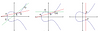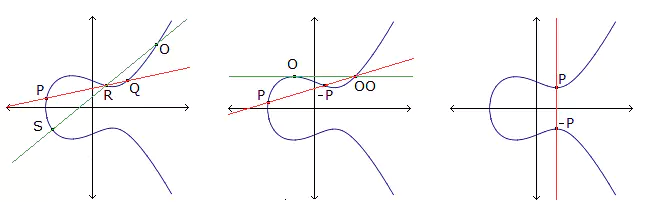# An $\mathbb{F}_{p^2}$-maximal Wiman's sextic and its automorphisms

### Journal article

M. Giulietti, M. Kawakita, Stefano Lia, M. Montanucci
2018

Semantic Scholar ArXiv
Cite

### Cite

APA
Giulietti, M., Kawakita, M., Lia, S., & Montanucci, M. (2018). An $\mathbb{F}_{p^2}$-maximal Wiman's sextic and its automorphisms.

Chicago/Turabian
Giulietti, M., M. Kawakita, Stefano Lia, and M. Montanucci. “An $\Mathbb{F}_{p^2}$-Maximal Wiman's Sextic and Its Automorphisms” (2018).

MLA
Giulietti, M., et al. An $\Mathbb{F}_{p^2}$-Maximal Wiman's Sextic and Its Automorphisms. 2018.

## Abstract

In 1895 Wiman introduced a Riemann surface $\mathcal{W}$ of genus $6$ over the complex field $\mathbb{C}$ defined by the homogeneous equation $\mathcal{W}:X^6+Y^6+Z^6+(X^2+Y^2+Z^2)(X^4+Y^4+Z^4)-12X^2 Y^2 Z^2=0$, and showed that its full automorphism group is isomorphic to the symmetric group $S_5$. The curve $\mathcal{W}$ was previously studied as a curve defined over a finite field $\mathbb{F}{p^2}$ where $p$ is a prime, and necessary and sufficient conditions for its maximality over $\mathbb{F}{p^2}$ were obtained. In this paper we first show that the result of Wiman concerning the automorphism group of $\mathcal{W}$ holds also over an algebraically closed field $\mathbb{K}$ of positive characteristic $p$, provided that $p \geq 7$. For $p=2,3$ the polynomial $X^6+Y^6+Z^6+(X^2+Y^2+Z^2)(X^4+Y^4+Z^4)-12X^2 Y^2 Z^2$ is not irreducible over $\mathbb{K}$, while for $p=5$ the curve $\mathcal{W}$ is rational and $Aut(\mathcal{W}) \cong PGL(2,\mathbb{K})$. We also show that the $\mathbb{F}{19^2}$-maximal Wiman's sextic $\mathcal{W}$ is not Galois covered by the Hermitian curve $\mathcal{H}{19}$ over $\mathbb{F}_{19^2}$.

Share